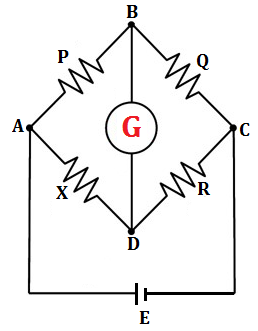# Wheatstone Bridge

It is an electrical circuit used for the measurement of resistance of the conductors. In Wheatstone bridge, there are total of four resistors in which three of them are known and one is unknown. The resistance of unknown resistance is calculated with reference to known three resistances.Wheatstone bridge

In figure, P, Q, X and R are four resistances in which the value of P, Q and R is known and X is unknown. A galvanometer G is connected across the junction B and D. A battery is connected to the circuit as shown in figure. When the value of P and Q is adjusted, R is varied in such a way that the current passes through the galvanometer becomes zero. At this condition, the galvanometer shows null deflection. At this condition:

$$\frac{\text{P}}{\text{Q}} = \frac{\text{X}}{\text{R}}$$

This is called balanced condition of Wheatstone bridge. In balanced condition, the junction B and D have same electric potential.

Do you like this article ? If yes then like otherwise dislike : 0